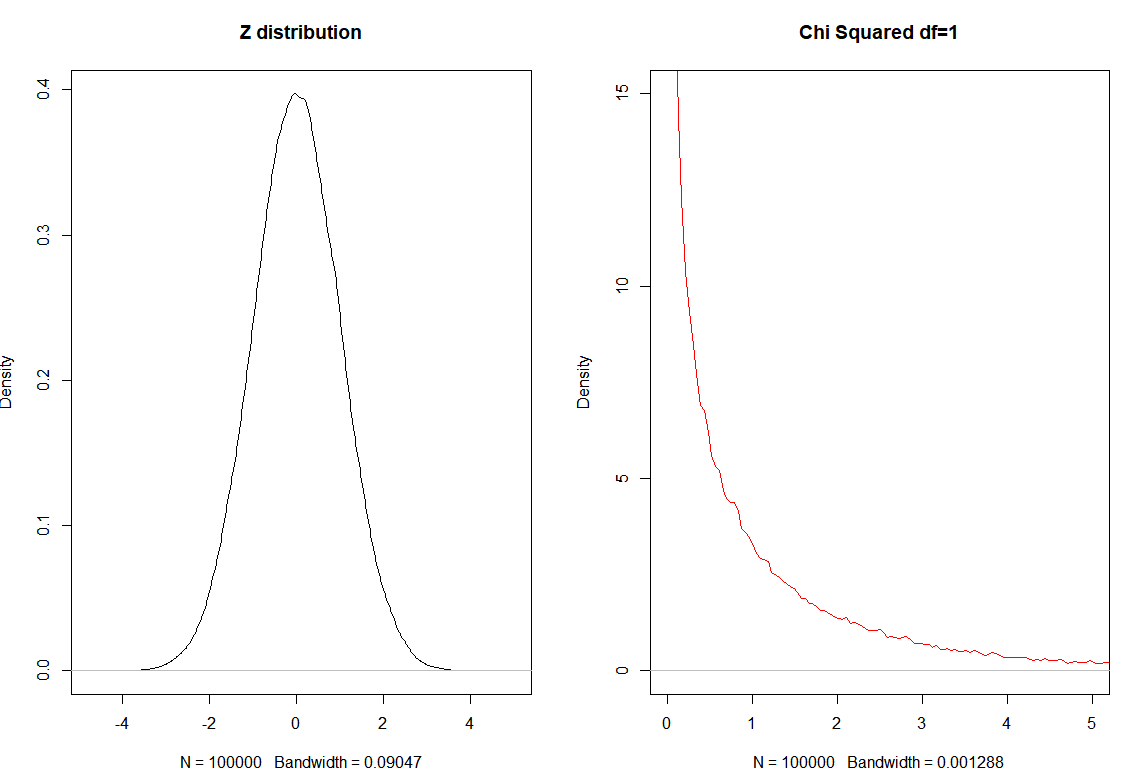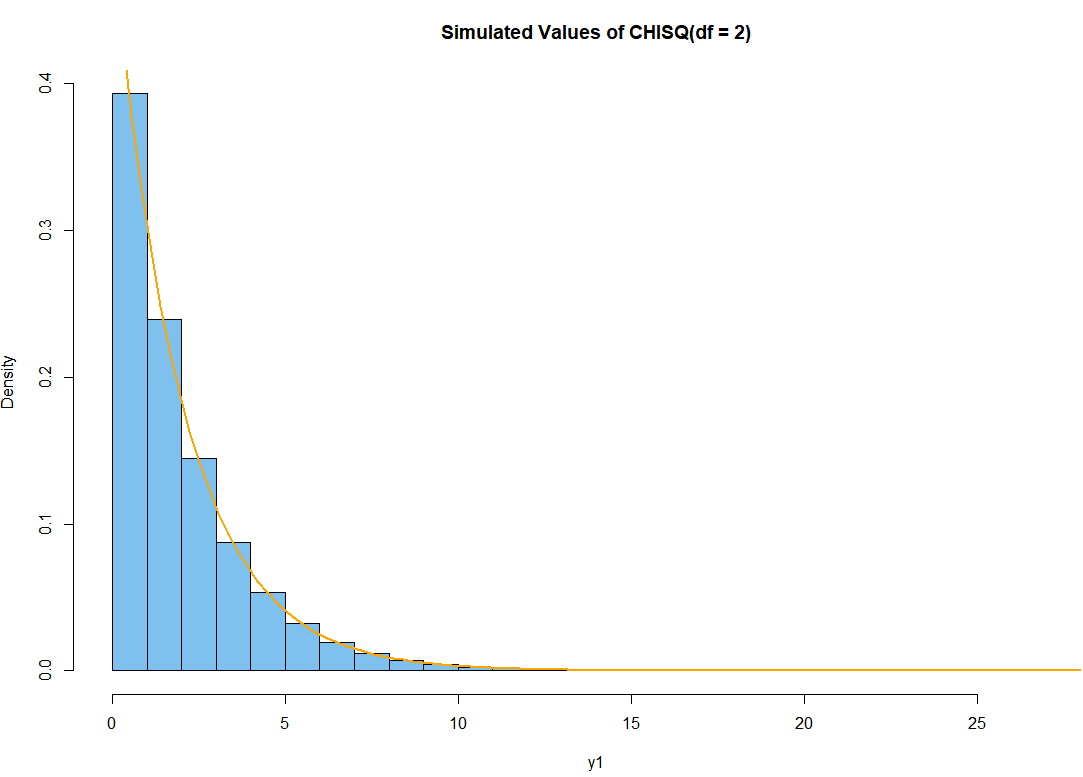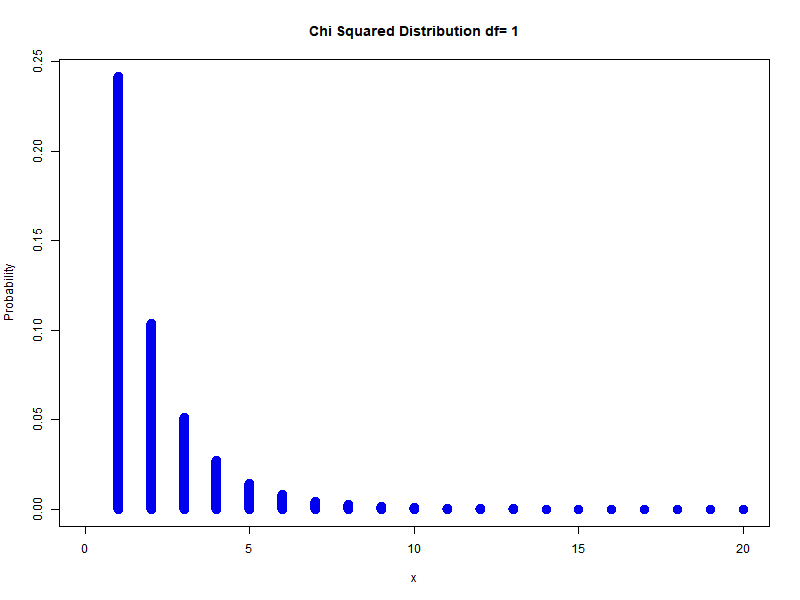# Chi Squared distribution in R| Examples

Discovered by Robert Helmert in 1875 chi-squared distribution is one of the most frequent distributions in statistics. It can be thought of as the “square” of a Z (standard normal distribution).

## Single degree of freedom

To create chi-squared distribution we start from Z-distribution.

$Z \sim \mathcal N(0,1)$

And write:

$Z_1^2 \sim \chi_1^2$
set.seed(0)
par(mfrow=c(1,2))
z = rnorm(10^5)
plot(density(z), main="Z distribution")
z2 = z^2
plot(density(z2, bw="SJ"),
main="Chi Squared df=1", col="red",
xlim=c(0,5), ylim=c(0,15))


Out:## Two degrees of freedom

How to get two degrees of freedom?

$Z_1^2 + Z_2^2 \sim \chi_2^2$

Simple we square two $Z$ distributions and sum them up to get what is called Chi-Squared distribution with two degrees of freedom.

R code simulation for $\chi_2^2$

set.seed(0)
x1 = rnorm(10^6);  x2 = rnorm(10^6)
y1 = x1^2 + x2^2
hdr = "Simulated Values of CHISQ(df = 2)"
hist(y1, prob=T, br=30, col="skyblue2", main=hdr)In general to get $k$ degrees of freedom:

$\sum_{i=1}^{n} Z_{i}^{2} \sim \chi_{n}^{2}$

Example: different degrees of freedom## PDF

Chi-Squared is actually gamma distribution with shape parameter $n/2$ and scale parameter $2$ is called the chi-square distribution with $n$ degrees of freedom. PDF is:

$f(x)=\frac{1}{2^{n / 2} \Gamma(n / 2)} x^{n / 2-1} e^{-x / 2}, \quad x \in(0, \infty)$

Example: Let’s show what happens if we square 10^5 numbers from $\mathcal N(1,2)$ distribution and check for the mean of these numbers.

set.seed(0)
x1 = rnorm(10^5, mean=1, sd=2)
y1 = x1^2
mean(y1)


Out:

5.013841


This mean is $\mu + \sigma^2$ in other words. For the case where we have $Z$ distributions it is only $\sigma^2$.

## Moments

For Variance $\mathbb V$ we have:

$\mathbb V(Z) =\mathbb E[Z^2] - ( \mathbb E[Z])^2$

Since $\mathbb E[Z]=0$ we have:

$\mathbb V(Z) =\mathbb E[Z^2]$

Finally, $\mathbb E[\chi_n]=n$, $\mathbb V(\chi_n) = 2n$

## Goodness of fit test

Example: 100 people were interviewed who is the GOAT of Basketball? The answers were: 43 for Michael Jordan, 36 LeBron James and 21 for Kobe Bryant. Is there a GOAT at 5% level of significance?

$H_0$: There is no GOAT

$H_A:$ There is GOAT

If we would show that there is no enough evidence for the $H_0$ we could show there is a GOAT.

Observations Expected
Jordan 43 100/3
LeBron 36 100/3
Briant 21 100/3
Total 100 100

We first need to determine the degrees of freedom for this case $df=2$

When working with tables $df$ is total number of rows - 1.

Now we need to find the critical 5% level of significance for the Chi Squared distribution with 2 degrees of freedom.

This is the same as asking the question at what value of $x$ we have the qchisq (quartile function) reaches the 95%.

qchisq(.95,2)


Out:

5.99


Now we need to calculate the sum in R

n=100 # total number of observations
e = n/3 # expected
obs = c(43, 36, 21) # observed values
sum((obs-e)^2/e)


Out

 2.8033333 0.2133333 4.5633333
 7.58


Because 7.58 > $\chi_{crit}^2$ = 5.99 we reject the hypothesis and show that preferred (GOAT) player exist.

The same can be achieved with this:

chisq.test(obs)


Out:

	Chi-squared test for given
probabilities

data:  obs
X-squared = 7.58, df = 2, p-value = 0.0226


Since p-value $<\alpha$ we reject $H_0$

Example: When will Novak Djokovic be GOAT if Federer and Nadal end their career with 20 GS?

tennis = c(20, 20, 35)
chisq.test(tennis)


Out:

	Chi-squared test for given
probabilities

data:  tennis
X-squared = 6, df = 2, p-value = 0.04979


We show that for $\alpha=0.05$ Novak needs 35 GS titles to become a GOAT.

Note in here we have trinomial distribution and expected values > 5 and we can use the Chi-squared test.

## Test For Independence

Example: Pearson Chi Square test also know as test of independence. Does the gender influence the favorite sport?

Male Female
Football 54 4
Volleyball 21 80

$H_0$ hypothesis: There is no evidence gender provides preference to sports.

$H_A$ hypothesis: Gender provides sport preference.

To resolve and answer the question fast we will use this R code:

data <- matrix(c(54, 4, 46, 39, 21, 80), ncol=2, byrow=TRUE)
data <- as.table(data)
data
chisq.test(data)


Out:

	Pearson's Chi-squared test

data:  data
X-squared = 78.134, df = 2, p-value < 2.2e-16


Because p-value < 0.05 we reject the hypothesis $H_0$ and approve the alternative hypothesis.

But how this even works? We create total columns or marginal frequencies.

Observed Male Female Total
Football 54 4 58
Volleyball 21 80 101
Total 121 123 244

Now we need to calculate the expected frequencies assuming the column row independence.

Expected Male Female Total
Football $\frac{58*121}{244}$ $\frac{58*123}{244}$ 58
Basketball $\frac{85*121}{244}$ $\frac{85*123}{244}$ 85
Volleyball $\frac{101*121}{244}$ $\frac{101*123}{244}$ 101
Total 121 123 244

Or after some calculus:

Expected Male Female Total
Football 28.76 29.24 58
Volleyball 50.08 50.91 101
Total 121 123 244

Now we need to calculate the sum (where $o$ means observed and $e$ expected value)

$$\chi^{2}=\sum \frac{(o-e)^{2}}{e}$$

Which lead us to the $\chi^{2}=78.134$ which is greater than critical $\chi_{crit}^{2}=5.99$ for 2 degrees of freedom. To calculate the degrees of freedom we use the formula $df=(c-1)(r-1)$ where $c$ is the number of columns and $r$ is number of rows.

We came to the exact same conclusion that gender influences the favorite sport in this case.

## De Moivre-Laplace theorem

You may asked yourself why Chi Squared distribution is used for Goodness of fit test?

Dealing with binomial distribution according to De Moivre-Laplace theorem when $n$ approaches $\infty$, having $p$ fixed the distribution of:

$\frac{X-n p}{\sqrt{n p(1-p)}} \sim z$

In here the mean is $np$ and standard deviation is $\sqrt{n p(1-p)}$.

So if the conditions are meat binomial or multinomial distributions in general case will convert to Z-distribution.

## Conclusion

To conclude the goodness of fit test is the same (or very similar) to the test for independence.

These tests are based on multinomial distributions and adhere to Z distribution when then the observed frequencies are big enough. Since we are interested in square metrics this is why we use Chi Square distribution.

tags: chisquared & category: r